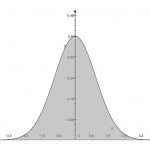# Computing ATM implied volatility analyticallyI recently had to compute ATM implied bpvol (or normal volatility) as well as Black volatility from an ATM option premium. Immediately, I looked for a library function which, more often than not, are written for the general case and make use of a solving routine. To my later irritation, I had completely forgotten that both bpvol and Black vol can be determined analytically (and trivially) from the ATM premium. So this note, is as much for my own interest as it is for anyone else’s interest.

Suppose the dynamics of a forward rate, F, follow the assumption of the normal model; that is,

$$dF=\nu dW$$

Then the forward value of a European option call option with strike $$K$$, expiring at time $$T$$ is,

$$C(K) = (F-K) N(d) + n(d) \nu\sqrt{T}$$
where, $$d=\frac{F-K}{\nu \sqrt{T}}$$ and $$n(x)=\frac{1}{\sqrt{2\pi}}e^{-x^2/2}$$. In the case of ATM, $$F=K$$, and the formula collapses to $$C(F)=\nu\sqrt{\frac{T}{2\pi}}$$, which is trivially invertible, so that,

$$\nu=C(F)\sqrt{\frac{2\pi}{T}}$$

Similarly, if one assumes the dynamics of a forward rate follow a lognormal process; that is,
$$dF=\sigma F dW$$

Then the one arrives at an implied Black vol of

$$\sigma = \frac{2}{\sqrt{T}} N^{-1} \left(\frac{1}{2}\left[\frac{C(F)}{F}+1\right]\right)$$
where, $$N^{-1}(x)$$ is the inverse of the cumulative normal distribution function. A good survey of accurate implementations is found in William Shaw’s article, Refinement of the Normal Quantile.

Stay informed with our FREE newsletter, subscribe here.

## 2 thoughts on “Computing ATM implied volatility analytically”

1.logos01 says:

This is why the Bachelier model is very elegant, the implied vol feels very natural and easy to grasp. I saw those formulae before in http://arxiv.org/pdf/0711.1272 (a good read on Bachelier & Black)‎. BTW the discus comments are crap to use.

1.Gary Kennedy says:

Disqus is gone.